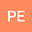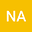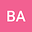Closed-form solution of a rational difference equation \$x_{n+1}=\frac{x_{n-(9 t+8)}}{1+\prod_{k=0}^{6} x_{n-(t+1) k-t} }
•••